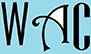## Trigonometry Question for Title 1 Schools: Non-Hypotenuse Question?

2) What is the value of the non-hypotenuse side of a right-angled triangle where the two other sides are 10 and 8?

Since we are looking for the value of the non-hypotenuse side, then the bigger of the two given side must be the hypotenuse “c”.

c2 = a2 + b2

Since we are looking for “a”, make “a” the subject of formula by moving “b” to the other side.  When you move to the other side, the signs MUST change.  Addition becomes subtraction, multiplication becomes division, and vice versa.

a2 = c2 – b2

a2 = 102 – 82

a2 = 100 – 64

a2 = 36

a = 6